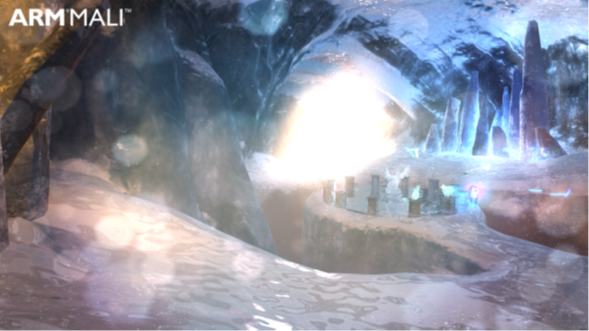## Dirty lens effect

In this section of the guide, we explain a simple implementation of a dirty lens effect that is suitable for mobile devices. A dirty lens effect can invoke a sense of drama and is often used together with a lens flare effect.

In the Ice Cave demo, the dirty lens effect is implemented by a small shader that uses a full screen quad laid over the top of the scene. The intensity of the quad, and hence the effect, is variable, with a value passed in from a script. The intensity of the quad is passed to it by a script.

The shader renders the quad that uses additive alpha blending at the very end, after all transparent geometry has been rendered.

The following code shows the `DirtyLensEffect.shader` code:

```half3 normalInWorld = half3(0.0,0.0,0.0);
half3 bumpNormal = UnpackNormal(tex2D(_BumpMapGlobal, input.tc));
half3x3 local2WorldTranspose = half3x3(input.tangentWorld,
input.bitangentWorld,
input.normalInWorld);
normalInWorld = normalize(mul(bumpNormal, local2WorldTranspose));
normalInWorld = normalInWorld*0.5 + 0.5;
return half4(normalInWorld,1.0);
```

This subsection describes the Dirty lens shader.

The following subshader tag instructs the full screen quad to be rendered after all opaque geometry, and after nine other transparent objects:

`Tags {"Queue" = "Transparent+10"}`

The shader uses the following command to deactivate depth buffer writing, which prevents the quad from occluding the geometry behind it:

`ZWrite Off`

At the blending stage, the output of the fragment shader is blended with the pixel color that is already in the frame buffer.

The shader specifies the additive blending type: `Blend One One`. In this blending type, the source and the destination factors are both `float4 (1.0, 1.0, 1.0, 1.0)`.

This type of blending is often used for particle systems when they represent effects like fire that are transparent and emit light.

The shader deactivates culling and ZTest to ensure that the dirty lens effect is always rendered. This means that the vertices of the quad are defined in viewport coordinates, so that no vertex transformations take place in the vertex shader.

Then the fragment shader only fetches the texel from the texture and applies a factor that is proportional to the intensity of the effect.

The following image shows the full screen quad texture for the dirty lens effect:The following image shows how the Dirty Lens effect looks in the Ice Cave demo. Specifically, when the camera is oriented to the sunlight that is coming from the entrance of the cave:#### Script implementation

A simple script creates the full screen quad and calculates the intensity factor that is passed to the fragment shader.

The following function creates the mesh of the quad in the Start function:

```void CreateQuadMesh()
{
Mesh mesh = GetComponent().mesh;
mesh.Clear();
mesh.vertices = new Vector3[] {new Vector3(-1, -1, 0), new Vector3(1, -1, 0), new Vector3(1, 1, 0), new Vector3(-1, 1, 0)};
mesh.uv = new Vector2[] {new Vector2(0, 0), new Vector2(1, 0), new Vector2(1, 1), new Vector2(0, 1)};
mesh.triangles = new int[] {0, 2, 1, 0, 3, 2};
mesh.RecalculateNormals();
//Increase bounds to avoid frustum clipping.
bigBounds.SetMinMax(new Vector3(-100, -100, -100), new Vector3(100, 100, 100));
mesh.bounds = bigBounds;
}
```

When the mesh is created, its bounds are incremented to guarantee that it is never frustum clipped from being outside the camera view. Therefore, you must set the size of the bounds according to the size of your scene.

The script calculates the intensity factor and passes it to the fragment shader. The intensity factor is based on the relative orientation of the camera-to-sun vector and the camera forward vector. The maximum intensity of the effect takes place when the camera is looking directly at the sun.

The following code shows the calculation of the intensity factor:

```Vector3 cameraSunVec = sun.transform.position - Camera.main.transform.position;
cameraSunVec.Normalize();
float dotProd = Vector3.Dot(Camera.main.transform.forward, cameraSunVec);
float intensityFactor = Mathf.Clamp(dotProd);
```